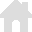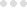vRigger Learning Center

This also applies to cable, chain, and webbing.

Gear that is anchored includes anchors, rocks, trees, tripods, trucks, etc.

A "bight" is a simple loop in a rope that does not cross itself.

A "bend" is a knot that joins two ropes together. Bends can only be attached to the end of a rope.

A "hitch" is a type of knot that must be tied around around another object.

"Descending devices" (e.g., ATCs, Brake Bar Racks, Figure 8s, Rescue 8s, etc) create friction as their primary purpose. The friction in descending devices is always considered when calculating forces.

The "Safety Factor" is the ratio between the gear's breaking strength and the maximum load applied to the gear (e.g., 5:1).

# Calculating Forces

See other force calculation topics.

Do one of the following to calculate forces:

• Choose Calculate Forces in the Calculations group on the Forces tab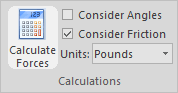• Press F8.

The calculated forces are valid until you modify the system (i.e., drag, edit, insert, or modify in the system). If you modify the system, you will need reselect Calculate Forces.

## Force Settings & Options

Options in the Calculations group on the Forces toolbar let you specify if friction and rope angles should be considered when calculating forces. You can also specify whether the results should be displayed in pounds, kilograms, or kilonewtons.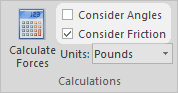Options in the Labels group on the Forces toolbar control where the forces are displayed.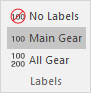Options in the Safety Factors group on the Forces toolbar control whether overload symbols are displayed.You can set the friction, strength, and weight of gear in the Forces section of the properties pane.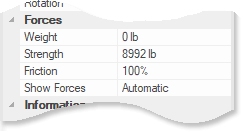If the results aren't what you expected, check out these troubleshooting tips.

REMINDER: The force calculations in vRigger are calculated based on a static, two-dimensional computer screen—the values can vary in the "real world." It is your responsibility to understand the strength of your rigging systems.